# dbscan

Density-based spatial clustering of applications with noise (DBSCAN)

Since R2019a

## Syntax

``idx = dbscan(X,epsilon,minpts)``
``idx = dbscan(X,epsilon,minpts,Name,Value)``
``idx = dbscan(D,epsilon,minpts,'Distance','precomputed')``
``[idx,corepts] = dbscan(___)``

## Description

example

````idx = dbscan(X,epsilon,minpts)` partitions observations in the n-by-p data matrix `X` into clusters using the DBSCAN algorithm (see Algorithms). `dbscan` clusters the observations (or points) based on a threshold for a neighborhood search radius `epsilon` and a minimum number of neighbors `minpts` required to identify a core point. The function returns an n-by-1 vector (`idx`) containing cluster indices of each observation.```

example

````idx = dbscan(X,epsilon,minpts,Name,Value)` specifies additional options using one or more name-value pair arguments. For example, you can specify `'Distance','minkowski','P',3` to use the Minkowski distance metric with an exponent of three in the DBSCAN algorithm.```

example

````idx = dbscan(D,epsilon,minpts,'Distance','precomputed')` returns a vector of cluster indices for the precomputed pairwise distances `D` between observations. `D` can be the output of `pdist` or `pdist2`, or a more general dissimilarity vector or matrix conforming to the output format of `pdist` or `pdist2`, respectively.```

example

````[idx,corepts] = dbscan(___)` also returns a logical vector `corepts` that contains the core points identified by `dbscan`, using any of the input argument combinations in the previous syntaxes.```

## Examples

collapse all

Cluster a 2-D circular data set using DBSCAN with the default Euclidean distance metric. Also, compare the results of clustering the data set using DBSCAN and k-Means clustering with the squared Euclidean distance metric.

Generate synthetic data that contains two noisy circles.

```rng('default') % For reproducibility % Parameters for data generation N = 300; % Size of each cluster r1 = 0.5; % Radius of first circle r2 = 5; % Radius of second circle theta = linspace(0,2*pi,N)'; X1 = r1*[cos(theta),sin(theta)]+ rand(N,1); X2 = r2*[cos(theta),sin(theta)]+ rand(N,1); X = [X1;X2]; % Noisy 2-D circular data set```

Visualize the data set.

`scatter(X(:,1),X(:,2))`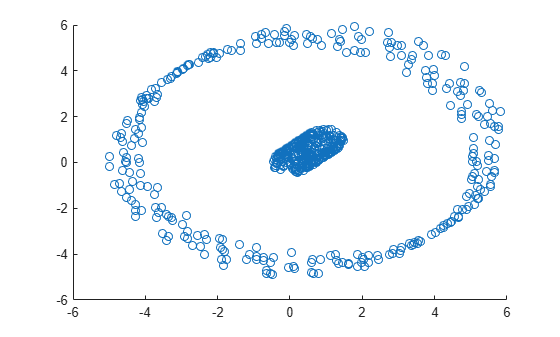The plot shows that the data set contains two distinct clusters.

Perform DBSCAN clustering on the data. Specify an `epsilon` value of 1 and a `minpts` value of 5.

`idx = dbscan(X,1,5); % The default distance metric is Euclidean distance`

Visualize the clustering.

```gscatter(X(:,1),X(:,2),idx); title('DBSCAN Using Euclidean Distance Metric')```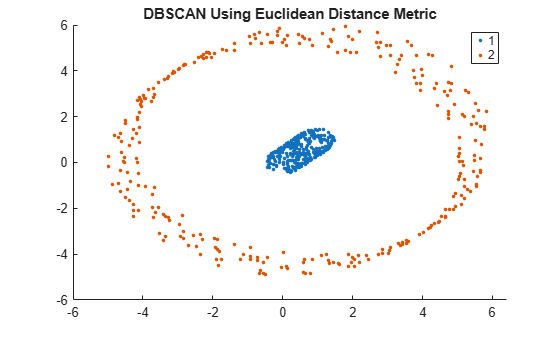Using the Euclidean distance metric, DBSCAN correctly identifies the two clusters in the data set.

Perform DBSCAN clustering using the squared Euclidean distance metric. Specify an `epsilon` value of 1 and a `minpts` value of 5.

`idx2 = dbscan(X,1,5,'Distance','squaredeuclidean');`

Visualize the clustering.

```gscatter(X(:,1),X(:,2),idx2); title('DBSCAN Using Squared Euclidean Distance Metric')```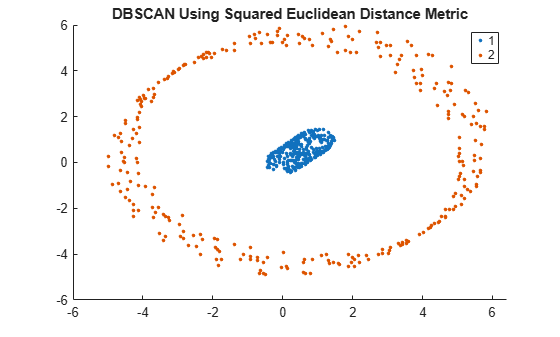Using the squared Euclidean distance metric, DBSCAN correctly identifies the two clusters in the data set.

Perform k-Means clustering using the squared Euclidean distance metric. Specify k = 2 clusters.

`kidx = kmeans(X,2); % The default distance metric is squared Euclidean distance`

Visualize the clustering.

```gscatter(X(:,1),X(:,2),kidx); title('K-Means Using Squared Euclidean Distance Metric')```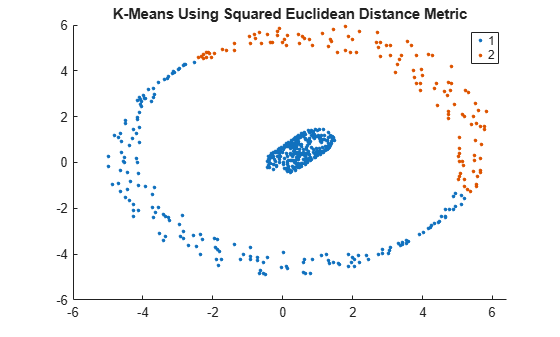Using the squared Euclidean distance metric, k-Means clustering fails to correctly identify the two clusters in the data set.

Perform DBSCAN clustering using a matrix of pairwise distances between observations as input to the `dbscan` function, and find the number of outliers and core points. The data set is a Lidar scan, stored as a collection of 3-D points, that contains the coordinates of objects surrounding a vehicle.

Load the x, y, z coordinates of the objects.

```load('lidar_subset.mat') loc = lidar_subset;```

To highlight the environment around the vehicle, set the region of interest to span 20 meters to the left and right of the vehicle, 20 meters in front and back of the vehicle, and the area above the surface of the road.

```xBound = 20; % in meters yBound = 20; % in meters zLowerBound = 0; % in meters```

Crop the data to contain only points within the specified region.

```indices = loc(:,1) <= xBound & loc(:,1) >= -xBound ... & loc(:,2) <= yBound & loc(:,2) >= -yBound ... & loc(:,3) > zLowerBound; loc = loc(indices,:);```

Visualize the data as a 2-D scatter plot. Annotate the plot to highlight the vehicle.

```scatter(loc(:,1),loc(:,2),'.'); annotation('ellipse',[0.48 0.48 .1 .1],'Color','red')```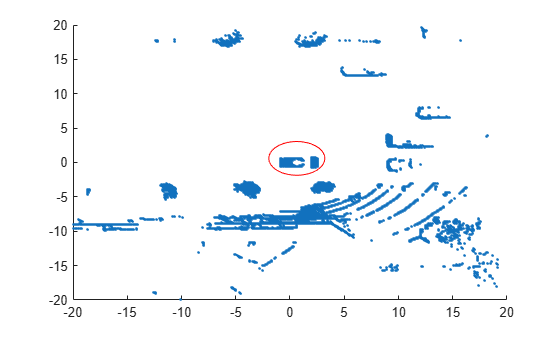The center of the set of points (circled in red) contains the roof and hood of the vehicle. All other points are obstacles.

Precompute a matrix of pairwise distances `D` between observations by using the `pdist2` function.

`D = pdist2(loc,loc);`

Cluster the data by using `dbscan` with the pairwise distances. Specify an `epsilon` value of 2 and a `minpts` value of 50.

`[idx, corepts] = dbscan(D,2,50,'Distance','precomputed');`

Visualize the results and annotate the figure to highlight a specific cluster.

```numGroups = length(unique(idx)); gscatter(loc(:,1),loc(:,2),idx,hsv(numGroups)); annotation('ellipse',[0.54 0.41 .07 .07],'Color','red') grid```As shown in the scatter plot, `dbscan` identifies 11 clusters and places the vehicle in a separate cluster.

`dbscan` assigns the group of points circled in red (and centered around (`3,–4`)) to the same cluster (group 7) as the group of points in the southeast quadrant of the plot. The expectation is that these groups should be in separate clusters. You can try using a smaller value of `epsilon` to split up large clusters and further partition the points.

The function also identifies some outliers (an `idx` value of `–1` ) in the data. Find the number of points that `dbscan` identifies as outliers.

`sum(idx == -1)`
```ans = 412 ```

`dbscan` identifies 412 outliers out of 19,070 observations.

Find the number of points that `dbscan` identifies as core points. A `corepts` value of `1` indicates a core point.

`sum(corepts == 1)`
```ans = 18446 ```

`dbscan` identifies 18,446 observations as core points.

See Determine Values for DBSCAN Parameters for a more extensive example.

## Input Arguments

collapse all

Input data, specified as an n-by-p numeric matrix. The rows of `X` correspond to observations (or points), and the columns correspond to variables.

Data Types: `single` | `double`

Pairwise distances between observations, specified as a numeric row vector that is the output of `pdist`, numeric square matrix that is the output of `pdist2`, logical row vector, or logical square matrix. `D` can also be a more general dissimilarity vector or matrix that conforms to the output format of `pdist` or `pdist2`, respectively.

For the aforementioned specifications, the following table describes the formats that `D` can take, given an input matrix `X` that has n observations (rows) and p dimensions (columns).

SpecificationFormat
Numeric row vector (output of `pdist(X)`)
• A row vector of length n(n – 1)/2, corresponding to pairs of observations in `X`

• Distances arranged in the order (2,1), (3,1), ..., (n,1), (3,2), ..., (n,2), ..., (n,n – 1))

Numeric square matrix (output of `pdist2(X,X)`)
• An n-by-n matrix, where `D(i,j)` is the distance between observations `i` and `j` in `X`

• A symmetric matrix having diagonal elements equal to zero

Logical row vector
• A row vector of length n(n – 1)/2, corresponding to pairs of observations in `X`

• A logical row vector with elements indicating distances that are less than or equal to `epsilon`

• Elements of `D` arranged in the order (2,1), (3,1), ..., (n,1), (3,2), ..., (n,2), ..., (n,n – 1))

Logical square matrix
• An n-by-n matrix, where `D(i,j)` indicates the distance between observations `i` and `j` in `X` that are less than or equal to `epsilon`

Note

If `D` is a logical vector or matrix, then the value of `epsilon` must be empty; for example, `dbscan(D,[],5,'Distance','precomputed')`.

Data Types: `single` | `double` | `logical`

Epsilon neighborhood of a point, specified as a numeric scalar that defines a neighborhood search radius around the point. If the epsilon neighborhood of a point contains at least `minpts` neighbors, then `dbscan` identifies the point as a core point.

The value of `epsilon` must be empty (`[]`) when `D` is a logical vector or matrix.

Example: `dbscan(X,2.5,10)`

Example: `dbscan(D,[],5,'Distance','precomputed')`, for a logical matrix or vector `D`

Data Types: `single` | `double`

Minimum number of neighbors required for a core point, specified as a positive integer. The epsilon neighborhood of a core point in a cluster must contain at least `minpts` neighbors, whereas the epsilon neighborhood of a border point can contain fewer neighbors than `minpts`.

Example: `dbscan(X,2.5,5)`

Data Types: `single` | `double`

### Name-Value Arguments

Specify optional pairs of arguments as `Name1=Value1,...,NameN=ValueN`, where `Name` is the argument name and `Value` is the corresponding value. Name-value arguments must appear after other arguments, but the order of the pairs does not matter.

Before R2021a, use commas to separate each name and value, and enclose `Name` in quotes.

Example: `dbscan(D,2.5,5,'Distance','precomputed')` specifies DBSCAN clustering using a precomputed matrix of pairwise distances `D` between observations, an epsilon neighborhood of `2.5`, and a minimum of `5` neighbors.

Distance metric, specified as the comma-separated pair consisting of `'Distance'` and a character vector, string scalar, or function handle, as described in this table.

ValueDescription
`'precomputed'`

Precomputed distances. You must specify this option if the first input to `dbscan` is a vector or matrix of pairwise distances `D`.

`'euclidean'`

Euclidean distance (default)

`'squaredeuclidean'`

Squared Euclidean distance. (This option is provided for efficiency only. It does not satisfy the triangle inequality.)

`'seuclidean'`

Standardized Euclidean distance. Each coordinate difference between observations is scaled by dividing by the corresponding element of the standard deviation, `S = std(X,'omitnan')`. Use `Scale` to specify another value for `S`.

`'mahalanobis'`

Mahalanobis distance using the sample covariance of `X`, `C = cov(X,'omitrows')`. Use `Cov` to specify another value for `C`, where the matrix `C` is symmetric and positive definite.

`'cityblock'`

City block distance

`'minkowski'`

Minkowski distance. The default exponent is 2. Use `P` to specify a different exponent, where `P` is a positive scalar value.

`'chebychev'`

Chebychev distance (maximum coordinate difference)

`'cosine'`

One minus the cosine of the included angle between points (treated as vectors)

`'correlation'`

One minus the sample correlation between points (treated as sequences of values)

`'hamming'`

Hamming distance, which is the percentage of coordinates that differ

`'jaccard'`

One minus the Jaccard coefficient, which is the percentage of nonzero coordinates that differ

`'spearman'`

One minus the sample Spearman's rank correlation between observations (treated as sequences of values)

`@distfun`

Custom distance function handle. A distance function has the form

```function D2 = distfun(ZI,ZJ) % calculation of distance ...```
where

• `ZI` is a `1`-by-`n` vector containing a single observation.

• `ZJ` is an `m2`-by-`n` matrix containing multiple observations. `distfun` must accept a matrix `ZJ` with an arbitrary number of observations.

• `D2` is an `m2`-by-`1` vector of distances, and `D2(k)` is the distance between observations `ZI` and `ZJ(k,:)`.

If your data is not sparse, you can generally compute distance more quickly by using a built-in distance instead of a function handle.

For definitions, see Distance Metrics.

When you use the `'seuclidean'`, `'minkowski'`, or `'mahalanobis'` distance metric, you can specify the additional name-value pair argument `'Scale'`, `'P'`, or `'Cov'`, respectively, to control the distance metrics.

Example: `dbscan(X,2.5,5,'Distance','minkowski','P',3)` specifies an epsilon neighborhood of `2.5`, a minimum of `5` neighbors to grow a cluster, and use of the Minkowski distance metric with an exponent of `3` when performing the clustering algorithm.

Exponent for the Minkowski distance metric, specified as the comma-separated pair consisting of `'P'` and a positive scalar.

This argument is valid only if `'Distance'` is `'minkowski'`.

Example: `'P',3`

Data Types: `single` | `double`

Covariance matrix for the Mahalanobis distance metric, specified as the comma-separated pair consisting of `'Cov'` and a symmetric, positive definite, numeric matrix.

This argument is valid only if `'Distance'` is `'mahalanobis'`.

Data Types: `single` | `double`

Scaling factors for the standardized Euclidean distance metric, specified as the comma-separated pair consisting of `'Scale'` and a numeric vector of positive values.

Each dimension (column) of `X` has a corresponding value in `'Scale'`; therefore, `'Scale'` is of length p (the number of columns in `X`). For each dimension of `X`, `dbscan` uses the corresponding value in `'Scale'` to standardize the difference between `X` and a query point.

This argument is valid only if `'Distance'` is `'seuclidean'`.

Data Types: `single` | `double`

## Output Arguments

collapse all

Cluster indices, returned as a numeric column vector. `idx` has n rows, and each row of `idx` indicates the cluster assignment of the corresponding observation in `X`. An index equal to `–1` indicates an outlier (or noise point).

Note

Cluster assignment using the DBSCAN algorithm is dependent on the order of observations. Therefore, shuffling the rows of `X` can lead to different cluster assignments for the observations. For more details, see Algorithms.

Data Types: `double`

Indicator for core points, returned as an n-by-1 logical vector indicating the indices of the core points identified by `dbscan`. A value of `1` in any row of `corepts` indicates that the corresponding observation in `X` is a core point. Otherwise, `corepts` has a value of `0` for rows corresponding to observations that are not core points.

Data Types: `logical`

collapse all

### Core Points

Core points in a cluster are points that have at least a minimum number of neighbors (`minpts`) in their epsilon neighborhood (`epsilon`). Each cluster must contain at least one core point.

### Border Points

Border points in a cluster are points that have fewer than the required minimum number of neighbors for a core point (`minpts`) in their epsilon neighborhood (`epsilon`). Generally, the epsilon neighborhood of a border point contains significantly fewer points than the epsilon neighborhood of a core point.

### Noise Points

Noise points are outliers that do not belong to any cluster.

## Tips

• For improved speed when iterating over many values of `epsilon`, consider passing in `D` as the input to `dbscan`. This approach prevents the function from having to compute the distances at every point of the iteration.

• If you use `pdist2` to precompute `D`, do not specify the `'Smallest'` or `'Largest'` name-value pair arguments of `pdist2` to select or sort columns of `D`. Selecting fewer than n distances results in an error, because `dbscan` expects `D` to be a square matrix. Sorting the distances in each column of `D` leads to a loss in the interpretation of `D` and can give meaningless results when used in the `dbscan` function.

• For efficient memory usage, consider passing in `D` as a logical matrix rather than a numeric matrix to `dbscan` when `D` is large. By default, MATLAB® stores each value in a numeric matrix using 8 bytes (64 bits), and each value in a logical matrix using 1 byte (8 bits).

• To select a value for `minpts`, consider a value greater than or equal to the number of dimensions of the input data plus one . For example, for an n-by-p matrix `X`, set `'minpts'` equal to p+1 or greater.

• One possible strategy for selecting a value for `epsilon` is to generate a k-distance graph for `X`. For each point in `X`, find the distance to the kth nearest point, and plot sorted points against this distance. Generally, the graph contains a knee. The distance that corresponds to the knee is typically a good choice for `epsilon`, because it is the region where points start tailing off into outlier (noise) territory .

## Algorithms

• DBSCAN is a density-based clustering algorithm that is designed to discover clusters and noise in data. The algorithm identifies three kinds of points: core points, border points, and noise points . For specified values of `epsilon` and `minpts`, the `dbscan` function implements the algorithm as follows:

1. From the input data set `X`, select the first unlabeled observation x1 as the current point, and initialize the first cluster label C to 1.

2. Find the set of points within the epsilon neighborhood `epsilon` of the current point. These points are the neighbors.

1. If the number of neighbors is less than `minpts`, then label the current point as a noise point (or an outlier). Go to step 4.

Note

`dbscan` can reassign noise points to clusters if the noise points later satisfy the constraints set by `epsilon` and `minpts` from some other point in `X`. This process of reassigning points happens for border points of a cluster.

2. Otherwise, label the current point as a core point belonging to cluster C.

3. Iterate over each neighbor (new current point) and repeat step 2 until no new neighbors are found that can be labeled as belonging to the current cluster C.

4. Select the next unlabeled point in `X` as the current point, and increase the cluster count by 1.

5. Repeat steps 2–4 until all points in `X` are labeled.

• If two clusters have varying densities and are close to each other, that is, the distance between two border points (one from each cluster) is less than `epsilon`, then `dbscan` can merge the two clusters into one.

• Every valid cluster might not contain at least `minpts` observations. For example, `dbscan` can identify a border point belonging to two clusters that are close to each other. In such a situation, the algorithm assigns the border point to the first discovered cluster. As a result, the second cluster is still a valid cluster, but it can have fewer than `minpts` observations.

 Ester, M., H.-P. Kriegel, J. Sander, and X. Xiaowei. “A density-based algorithm for discovering clusters in large spatial databases with noise.” In Proceedings of the Second International Conference on Knowledge Discovery in Databases and Data Mining, 226-231. Portland, OR: AAAI Press, 1996.